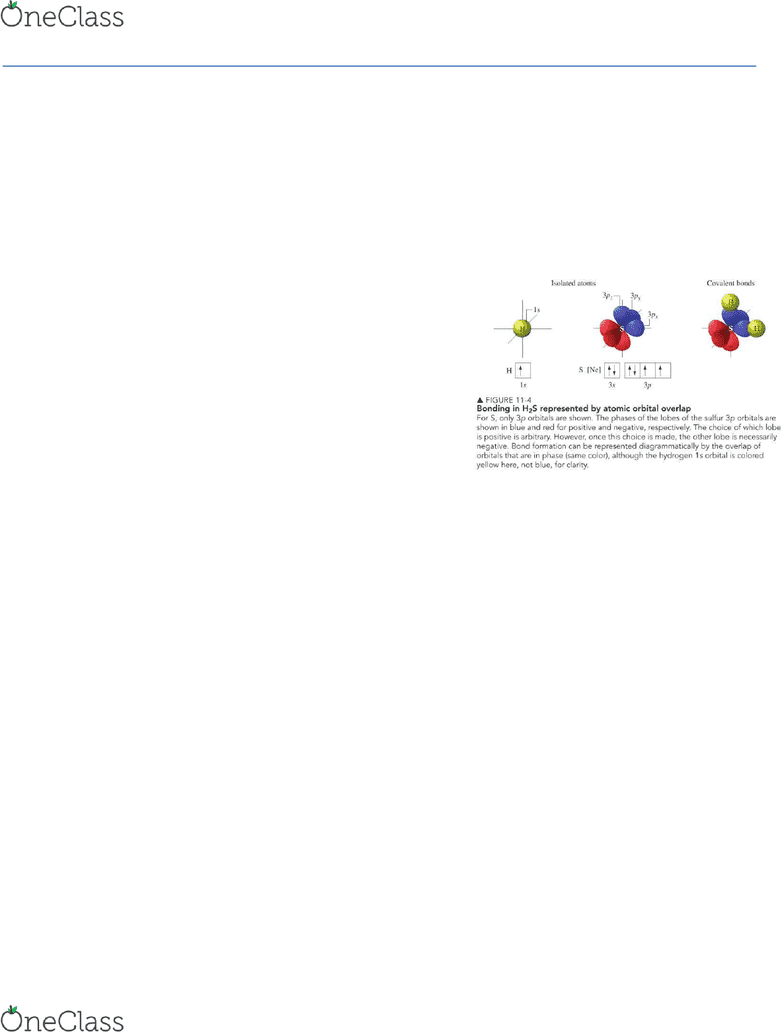Textbook Notes (280,000)
CA (170,000)
Queen's (4,000)
CHEM (40)
Chapter 11.5 and 11.2

# CHEM 112 Chapter Notes - Chapter 11.5 and 11.2: Molecular Orbital Theory, Pauli Exclusion Principle, Atomic Orbital

Department
Chemistry
Course Code
CHEM 112
Professor
Gang Wu
Chapter
11.5 and 11.2

This preview shows half of the first page. to view the full 3 pages of the document.Chemistry Week 5
11-2
Introduction to the valence bond method
AOs= atomic orbitals
The region of high electron probability in a hydrogen atom is the 1s orbital
As the two hydrogen atoms approach each other their atomic orbitals overlap and a covalent bond can be formed
Bond formation involves an increase in electron probability density between two positively charged nuclei
Description of covalent bonds formed in terms of atomic orbital overlap is called valence bond theory
The creation of a covalent bond in the valence bond method is normally based on the overlap of half filled orbitals
Gives a localized electron model of bonding
o Core electrons and lone pair electrons retain the same orbital locations as in the separated atoms but the bonding electrons do not
o Instead they are described by an electron probability density that includes the region of orbital overlap and both nuclei
Problems with valence bond theory
o Elements that hybridize
11-5
Lewis structures, VSEPR theory and valence bond theory are satisfactory to explain
covalent bonds and molecular structures
None of these methods explain why oxygen is paramagnetic or why H2+ is a stable
species
Molecular orbital theory
Unlike atomic orbitals which are centered on a single nucleus, molecular orbitals
are defined with respect to all nuclei
Molecular orbital calculation for F2
o MOs are obtained for the analysis of the total wave function describing
the F2 molecule at its lowest energy state
o Solve the Schrödinger equation to determine the total wave function
o There are 10 MOs and are distributed around both nuclei
o Rationalize the number and shapes of MOs through the LCAO
o Linear combination of atomic orbitals
Method is based on the idea that because a molecule is composed of atoms, the atomic orbitals of those atoms can be used
as the basis of a method for describing how each electron in a molecule interacts with all nuclei simultaneously
LCAO
o Each MO can be represented mathematically as a linear combination of AOs
We imagine that AOs are transformed into a set of molecular orbitals when the atoms combine to form the molecule
o The total number of MOs is equal to the total number of AOs
Electrons in the ground state F atom are described by fine atomic orbitals (1s, 2s, 2px, 2py, 2pz)
Thus the electrons for two isolated F atoms are described by a total of 10 orbitals
10 AOs combine to form 10 MOs
o MOs can be classified as bonding, antibonding or nonbonding
This classification is made considering the electron density, which is the square of the wave function representing a MO, in
the nuclear region
Bonding orbital s will have electron density between the nuclei
Antibonding orbitals will have a node between the nuclei
Non bonding orbitals will typically consist of AOs isolated on one or more atoms and neither add to nor detract from bond
formation
o The electron configuration for a molecule is obtained by placing the electrons into the MOs in a particular order
Rules like the Pauli exclusion principle and Hunds rule also apply to molecules
Combination of two hydrogens to form H2
o The molecular orbital resulting from the addition of the 1s orbitals is a bonding molecular orbital
There is an electron density between the nuclei that the molecular orbital has a cylindrical shape
This bonding molecular orbital is given the label and is lower in energy than the 1s atomic orbitals
o The molecular orbital resulting from the subtraction of the 1a orbitals is an antibonding molecular orbital
This orbital is described as antibonding because the electron density is zero at a point between the nuclei
The antibonding orbital results from the subtraction of two 1s orbitals and is thus labeled as 
The is higher in energy than the 1s atomic orbitals
Stable molecular species has more electrons in bonding orbitals than in antibonding orbitals
If the excess of bonding over antibonding electrons is two, this corresponds to a single covalent bond in lewis theory
In molecular orbital theory we say that bond order is 1
Bond order is one half the difference between the number of bonding and antibonding electrons
find more resources at oneclass.com
find more resources at oneclass.com